##### Differential Equations For Dummies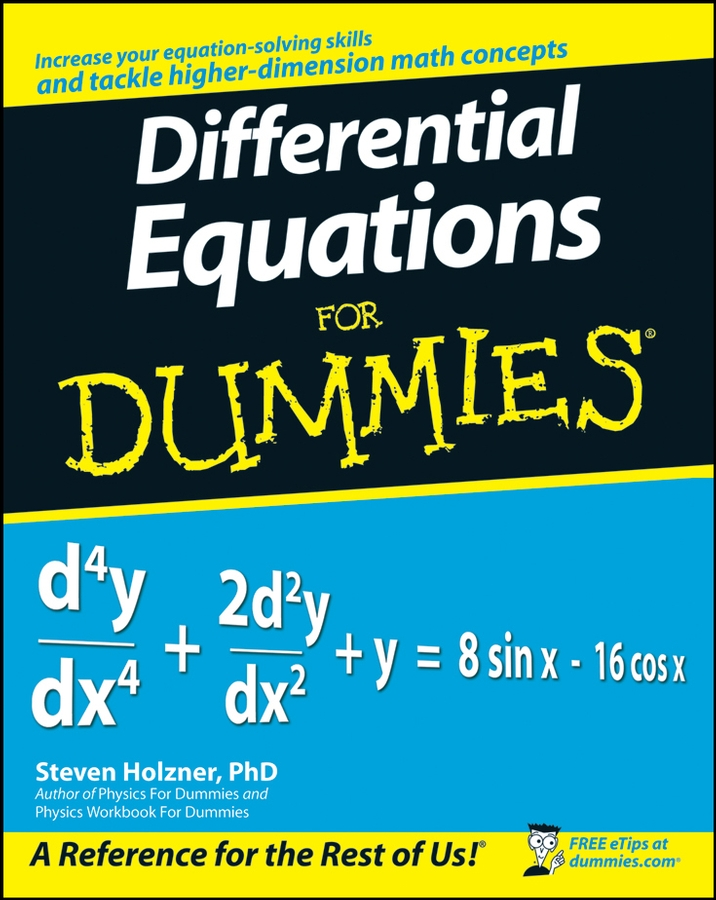You can approximate the area under a curve by using right sums. This method works just like the left sum method except that each rectangle is drawn so that its right upper corner touches the curve instead of its left upper corner.

For example, estimate the area under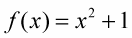from 0 to 3 by using right rectangles, as shown in this figure.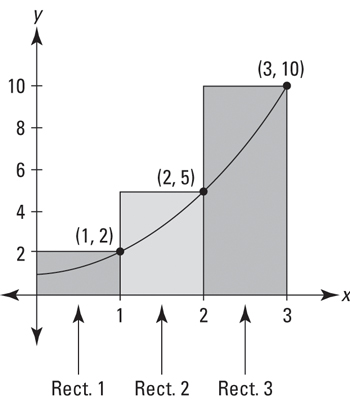The heights of the three rectangles are given by the function values at their right edges: f(1) = 2, f(2) = 5, and f(3) = 10. Each rectangle has a width of 1, so the areas are 2, 5, and 10, which total 17. This approximation gives you an overestimate of the actual area under the curve.

The more rectangles you create between 0 and 3, the more accurate your estimate will be. Here's the Right Rectangle Rule: You can approximate the exact area under a curve between a and b,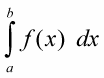with a sum of right rectangles given by this formula: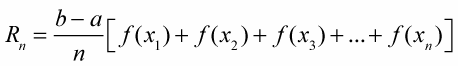Where, n is the number of rectangles,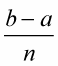is the width of each rectangle, and the function values are the heights of the rectangles.

Now if you compare this formula to the one for a left rectangle sum, you get the complete picture about those subscripts. The two formulas are the same except for one thing. Look at the sums of the function values in both formulas. The right sum formula has one value,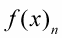that the left sum formula doesn’t have, and the left sum formula has one value,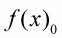that the right sum formula doesn’t have. All the function values between those two appear in both formulas. You can get a better handle on this by comparing the three left rectangles from the below figure to the three right rectangles from the above figure.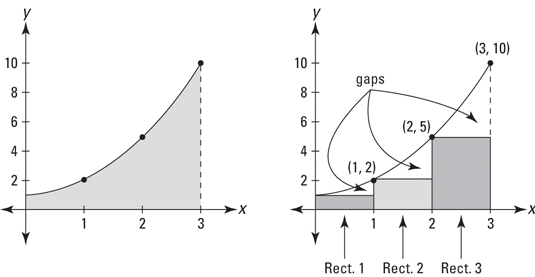For the left sum, you can find the areas and totals using the following formula: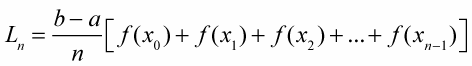So, three left rectangles add up to: 1 + 2 + 5 = 8

For the right sum, you can use the following formula:So, three right rectangles add up to: 2 + 5 + 10 = 17

The sums of the areas are the same except for the left-most left rectangle and the right-most right rectangle. Both sums include the rectangles with areas 2 and 5. If you look at how the rectangles are constructed, you can see that the second and third rectangles in the second figure are the same as the first and second rectangles in the first figure.

One last thing on this. The difference between the right rectangle total area (17) and the left rectangle total area (8) — that’s 17 minus 8, or 9, in case you love calculus but don’t have the basic subtraction thing down yet — comes from the difference between the areas of the two “end” rectangles just discussed (10 minus 1 is also 9). All the other rectangles are a wash, no matter how many rectangles you have.